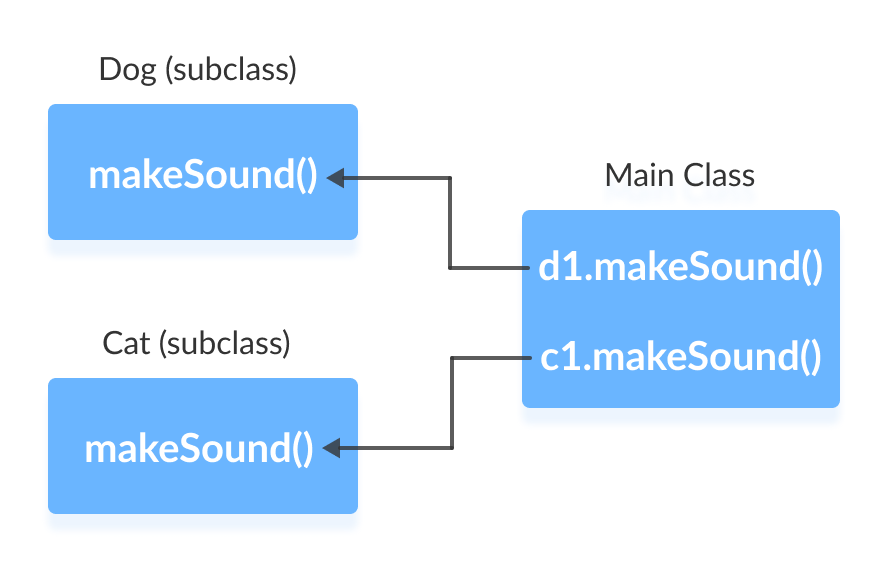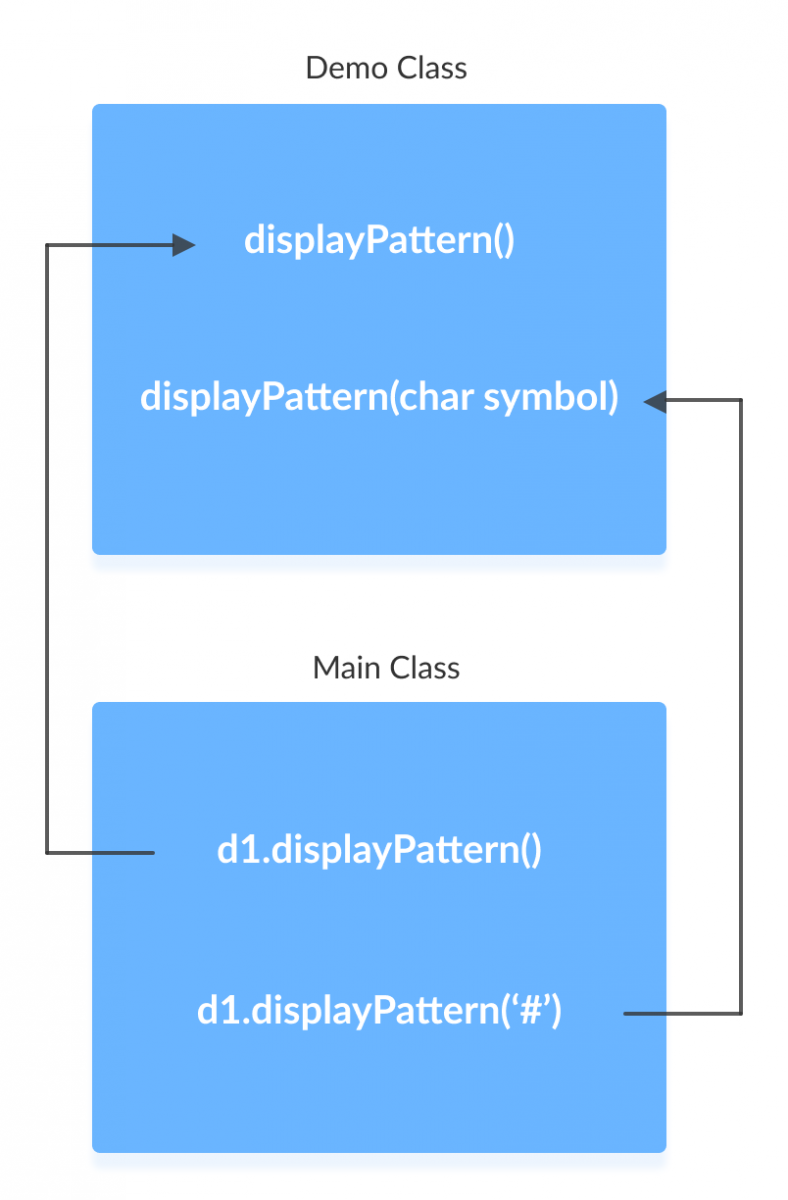# Java Polymorphism

In this tutorial, we will learn about polymorphism, different types of polymorphism and how to implement them in Java with the help of examples.

Polymorphism is an important concept of object-oriented programming. It simply means more than one form. That is, the same entity (method or operator or object) behaves differently in different scenarios. For example,

The `+` operator in Java is used to perform two specific functions. When it is used with numbers (integers and floating-point numbers), it performs addition.

``````int a = 5;
int b = 6;
int sum = a + b;     //  Output = 11
``````

And when we use `+` operator with strings, it performs string concatenation. For example,

``````String firstName = "abc ";
String lastName = "xyz";
name = firstName + lastName;     //  Output = abc xyz
``````

## Types of Polymorphism

In Java, Polymorphism can be divided into two types:

• Run-time Polymorphism
• Compile-time Polymorphism

## Run-time Polymorphism

In Java, run-time polymorphism can be achieved through method overriding.

Suppose the same method is created in the superclass and its subclasses. In this case, the method that will be called depends upon the object used to call the method. For example,

### Example 1: Method Overriding

``````abstract class Animal {
public abstract void makeSound();
}

class Dog extends Animal {
@override
public void makeSound() {
System.out.println("Bark bark..");
}
}

class Cat extends Animal {
@override
public void makeSound() {
System.out.println("Meow meow..");
}
}

class Main {
public static void main(String[] args) {
Dog  d1 = new Dog();
d1.makeSound();

Cat c1 = new Cat();
c1.makeSound();
}
}
``````

When we run the program, the output will be:

```Bark bark…
Meow-meow...
```

To know how method overriding works visit Method Overriding in Java.

In the above example, the method `makeSound()` has different implementations in two different classes. When we run the program,

• the expression `d1.makeSound()` will call the method of Dog class. It is because d1 is an object of the Dog class.
• the expression `c1.makeSound()` will call the method of Cat class. It is because c1 is an object of the cat class.The method that will be called is determined during the execution of the program. Hence, method overriding is a run-time polymorphism.

## Compile-time Polymorphism

In a Java class, we can create methods with the same name if they differ in parameters. For example,

``````void func() { ... }
void func(int a) { ... }
float func(double a) { ... }
float func(int a, float b) { ... }
``````

``````class Demo {
public void displayPattern(){
for(int i = 0; i < 10; i++) {
System.out.print("*");
}
}

public void displayPattern(char symbol) {
for(int i = 0; i < 10; i++) {
System.out.print(symbol);
}
}
}

class Main {
public static void main(String[] args) {
Demo d1 = new Demo();
d1.displayPattern();
System.out.println("\n");
d1.displayPattern('#');
}
}

``````

When we run the program, the output will be:

```**********
##########
```

In the above program, the `displayPattern()` method is overloaded.

• If we call the method without passing any arguments, a pattern of `*` is created.
• If we call the method by passing a character as an argument, a pattern of that character is created.• In the case of method overriding, methods should be inside different classes. Whereas, in the case of method overloading, methods should be inside the same class.
• Method overriding is performed at run-time whereas method overloading is performed at compile-time.

Some operators in Java behave differently with different operands. For example,

• `+` operator is overloaded to perform numeric addition as well as string concatenation, and
• operators like `&`, `|`, and `!` are overloaded for logical and bitwise operations.

Let’s see how an operator is overloaded in Java.

The `+` operator in Java is used to perform two specific functions. When it is used with numbers (integers and floating-point numbers), it performs addition. For example,

``````int a = 5;
int b = 6;
int sum = a + b;     //  Output = 11
``````

And when we use `+` operator with strings, it performs string concatenation. For example,

``````String firstName = "abc ";
String lastName = "xyz";
name = firstName + lastName;     //  Output = abc xyz
``````

In languages like C++, we can define operators to work differently for different operands. However, Java doesn’t support user-defined operator overloading.

### Why Polymorphism?

Polymorphism allows us to create consistent code. For example,

Suppose we need to render a circle and a square. To do so, we can create a Polygon class and inherited two subclasses Circle and square from it. In this case, it makes sense to create a method having the same name `render()` in both these subclasses rather creating methods with different names.

In our method overloading example, we have used the same method name `displayPattern()` to display two different patterns for consistency.

The` print()` method in Java is also an example of Polymorphism (method overloading). The same method is used to print values of different types like `char`, `int`, `String` etc. We can also use the same method to print multiple values at once.

## Polymorphic Variables

In Java, object variables (instance variables) represent the behavior of polymorphic variables. It is because object variables of a class can refer to objects of its class as well as objects of its subclasses. For example,

``````class Animal {
public void displayInfo() {
System.out.println("I am an animal.");
}
}

class Dog extends Animal {
@override
public void displayInfo() {
System.out.println("I am a dog.");
}
}

class Main {
public static void main(String[] args) {

// declaration of object variable a1 of the Animal class
Animal a1;

// object creation of the Animal class
a1 = new Animal();
a1.displayInfo();
// object creation of the dog class
a1 = new Dog();
a1.displayInfo();
}
}
``````

When we run the program, the output will be:

```I am an animal.
I am a dog.
```

In the above example, we have created an object variable a1 of the Animal class. Here, a1 is a polymorphic variable.

It is because,

• In statement `a1 = new Animal()`, a1 refers to the object of the Animal class.
• In statement `a1 = new Dog()`, a1 refers to the object of the Dog class.

This is an example of upcasting in Java. Visit upcasting and downcasting in Java to learn more.